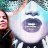# Percentage Change since March Lows Watchlist Column#### BenTen

Staff
VIP
I've recently wondered which stocks in my watchlist have the greatest and lowest percentage change since the stock market "crash" in March 2020. Here's a watchlist column and label to help you identify the stock percentage change since the pandemic panic low on March 23.### Watchlist column

Code:
``````# Stocks Percentage Change since March lows
# Assembled by BenTen at UseThinkScript.com

def close_value = close(period = AggregationPeriod.DAY);
def date1 = GetYYYYMMDD() == 20200323;
def date2 = GetYYYYMMDD() == GetYYYYMMDD();
def prev = if date1 then close_value else prev;
def current = if date2 then close_value else current;

def test = Round((current - prev) / prev);

For your watchlist column, be sure to set the aggregation period to D for Daily.

Code:
``````# Stocks Percentage Change since March lows
# Assembled by BenTen at UseThinkScript.com
def close_value = close(period = AggregationPeriod.DAY);
input source = 20200323;
def date1 = GetYYYYMMDD() == source;
def date2 = GetYYYYMMDD() == GetYYYYMMDD();
def prev = if date1 then close_value else prev;
def current = if date2 then close_value else current;

def test = Round((current - prev) / prev);
#AddLabel(yes, Concat("Change since March's low = %", test), color.orange);

AddLabel(1, "Change since March's low = " + AsPercent(test), color.orange);``````Need % percentage change in Stochastic Questions 2